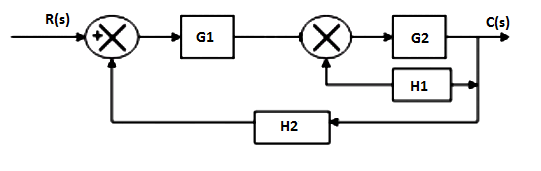# Block diagram Algebra Objective Questions

Block diagram Algebra Objective Questions

1. Consider the block diagram shown below:If the transfer function of the system is given by T(s)=G1G2+G2G3/1+X. Then X is:

a) G2G3G4
b) G2G4
c) G1G2G4
d) G3G4

Explanation: Use the technique of making two different block diagram by dividing two summers and use the approaches of shifting take off point and blocks.

2. For the block diagram given in the following figure, the expression of C/R is:a) G1G2G3/1-G2G1
b) G1G2/1-G1G2G3
c) G1G2G3/1-G1G2G3
d) G1G2/G3(1-G1G2)

Explanation: Block diagram is being converted into signal flow graphs by considering each take off point as a node and each forward transfer function as forward gain.

3. The transfer function from D(s) to Y(s) is :a) 2/3s+7
b) 2/3s+1
c) 6/3s+7
d) 2/3s+6

Explanation: Y(s)/D(s)=2/3s+1/1+3*(2/3s+1)=2/3s+7.

4. The closed loop gain of the system shown in the given figure is :a) -9/5
b) -6/5
c) 6/5
d) 9/5

Explanation: C(s)/R(s)=-3/1+3/2=-6/5.

5. The advantage of block diagram representation is that it is possible to evaluate the contribution of each component to the overall performance of the system.

a) True
b) False

Explanation: The advantage of the block diagram is that it is possible to get the contribution of each block to the overall performance of the system.

6. The overall transfer function from block diagram reduction for cascaded blocks is :

a) Sum of individual gain
b) Product of individual gain
c) Difference of individual gain
d) Division of individual gain

Explanation: Gain of block get multiplied when they are cascaded where cascaded means that the blocks are in series combination with no summer in between.

7. The overall transfer function of two blocks in parallel are :

a) Sum of individual gain
b) Product of individual gain
c) Difference of individual gain
d) Division of individual gain

Explanation: The gains get added as the blocks are connected in parallel with the summer in between and they are connected with the same sign.

8. Transfer function of the system is defined as the ratio of Laplace output to Laplace input considering initial conditions________

a) 1
b) 2
c) 0
d) infinite

Explanation: By definition transfer function is the ratio of the laplace output to the input but the initial conditions mainly the stored energy is zero.

9. In the following block diagram, G1=10/s G2=10/s+1 H1=s+3, H2=1. The overall transfer function is given by :a) 10/11s2+31s+10
b) 100/11s2+31s+100
c) 100/11s2+31s+10
d) 100/11s2+31s

Explanation: C/R=G2G1/1+G2H2+G1G2H2
C/R=100/11s2+31s+100.

10. Oscillations in output response is due to :

a) Positive feedback
b) Negative feedback
c) No feedback
d) None of the mentioned